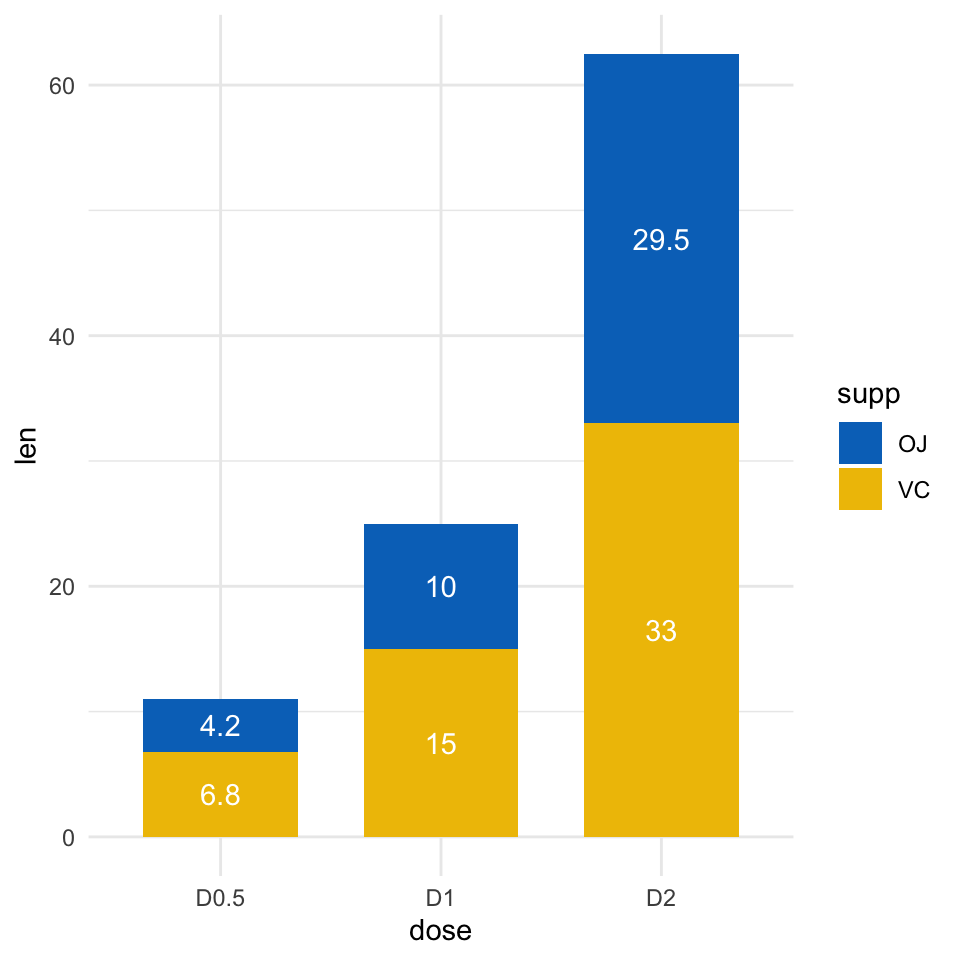# How to Create a GGPlot Stacked Bar Chart#### How to Create a GGPlot Stacked Bar Chart

This tutorial describes how to create a ggplot stacked bar chart. You will also learn how to add labels to a stacked bar plot.

#### Related Book

GGPlot2 Essentials for Great Data Visualization in R

## Prerequisites

Load required packages and set the theme function theme_minimal() as the default theme:

library(dplyr)   # For data manipulation
library(ggplot2) # For data visualization
theme_set(theme_minimal())

## Data preparation

Data derived from ToothGrowth data sets are used. ToothGrowth describes the effect of Vitamin C on tooth growth in Guinea pigs. Three dose levels of Vitamin C (0.5, 1, and 2 mg) with each of two delivery methods [orange juice (OJ) or ascorbic acid (VC)] are used :

df <- data.frame(
supp = rep(c("VC", "OJ"), each = 3),
dose = rep(c("D0.5", "D1", "D2"), 2),
len = c(6.8, 15, 33, 4.2, 10, 29.5)
)

head(df)
##   supp dose  len
## 1   VC D0.5  6.8
## 2   VC   D1 15.0
## 3   VC   D2 33.0
## 4   OJ D0.5  4.2
## 5   OJ   D1 10.0
## 6   OJ   D2 29.5
• len : Tooth length
• dose : Dose in milligrams (0.5, 1, 2)
• supp : Supplement type (VC or OJ)

## Basic plots

p <- ggplot(df, aes(x = dose, y = len))+
geom_col(aes(fill = supp), width = 0.7)
p4 steps required to compute the position of text labels:

• Group the data by the dose variable
• Sort the data by dose and supp columns. As stacked plot reverse the group order, supp column should be sorted in descending order.
• Calculate the cumulative sum of len for each dose category. Used as the y coordinates of labels. To put the label in the middle of the bars, we’ll use cumsum(len) - 0.5 * len.
• Create the bar graph and add labels
# Arrange/sort and compute cumulative summs
library(dplyr)
df2 <- df %>%
group_by(dose) %>%
arrange(dose, desc(supp)) %>%
mutate(lab_ypos = cumsum(len) - 0.5 * len)
df2
## # A tibble: 6 x 4
## # Groups:   dose 
##   supp  dose    len lab_ypos
##   <fct> <fct> <dbl>    <dbl>
## 1 VC    D0.5    6.8      3.4
## 2 OJ    D0.5    4.2      8.9
## 3 VC    D1     15        7.5
## 4 OJ    D1     10       20
## 5 VC    D2     33       16.5
## 6 OJ    D2     29.5     47.8
# Create stacked bar graphs with labels
p <- ggplot(data = df2, aes(x = dose, y = len)) +
geom_col(aes(fill = supp), width = 0.7)+
geom_text(aes(y = lab_ypos, label = len, group =supp), color = "white")
p## Customized bar plots

Use the function scale_fill_manual() to set manually the bars border line colors and area fill colors.

p + scale_fill_manual(values = c("#0073C2FF", "#EFC000FF"))•Andrea

Thank you so much, this is the first instruction for stacked plots that easily works!

•Kassambara

Thank you for the positive feedback, highly appreciated!

•Luca modritch

Easy to understand and well-explained instructions ! Thank you Kassambara